## Conformal Solution

By letting, the Real and Imaginary Parts of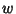must satisfy the Cauchy-Riemann Equations and Laplace's Equation, so they automatically provide a scalar Potential and a so-called stream function.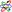If a physical problem can be found for which the solution is valid, we obtain a solution--which may have been very difficult to obtain directly--by working backwards. Let(1)

the Real and Imaginary Parts then give(2)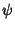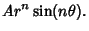(3)

For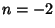,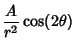(4)(5)

which is a double system of Lemniscates (Lamb 1945, p. 69). For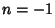,(6)(7)

This solution consists of two systems of Circles, andis the Potential Function for two Parallel opposite charged line charges (Feynman et al. 1989, §7-5; Lamb 1945, p. 69). For,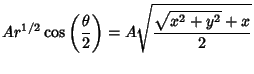(8)(9)gives the field near the edge of a thin plate (Feynman et al. 1989, §7-5). For,(10)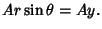(11)

This is two straight lines (Lamb 1945, p. 68). For,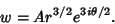(12)gives the field near the outside of a rectangular corner (Feynman et al. 1989, §7-5). For,(13)(14)(15)

These are two Perpendicular Hyperbolas, andis the Potential Function near the middle of two point charges or the field on the opening side of a charged Right Angle conductor(Feynman 1989, §7-3).